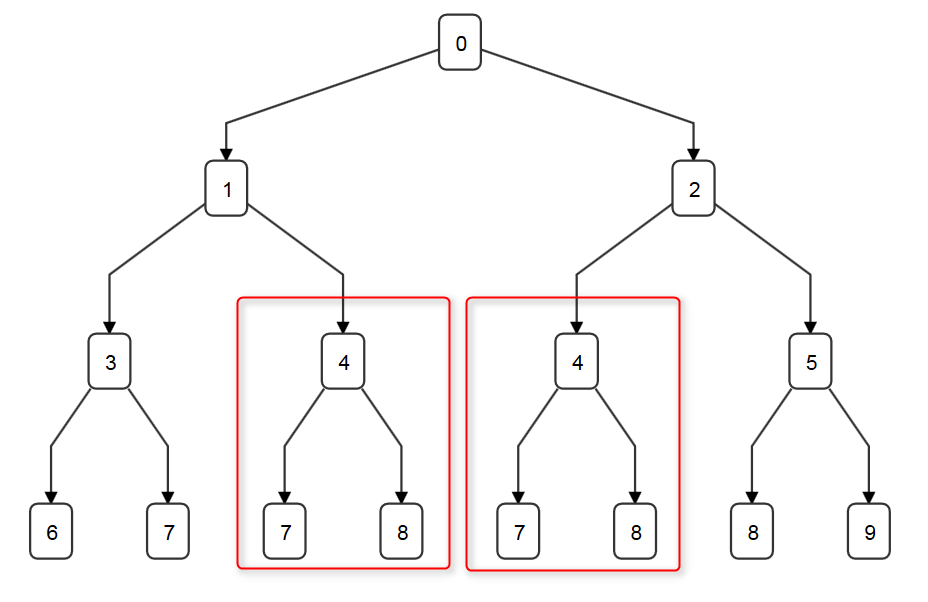# 120. Triangle

## 题目

Given a triangle, find the minimum path sum from top to bottom. Each step you may move to adjacent numbers on the row below.

For example, given the following triangle

[
,
[3,4],
[6,5,7],
[4,1,8,3]
]


The minimum path sum from top to bottom is 11 (i.e., 2 + 3 + 5 + 1 = 11).

Note:

Bonus point if you are able to do this using only O(n) extra space, where n is the total number of rows in the triangle.

## 思路

     ,
[1,2],
[3,4,5],
[6,7,8,9]dp算法：

dp[row][i]: 第row层第i个元素到达底层所需的最短路径

## 实现

 1     public int minimumTotal(List<List<Integer>> triangle) {
2         int N = triangle.size();
3         int[] min = new int[N];
4         List<Integer> lastRow = triangle.get(N - 1);
5         for(int i = 0; i < N; i++)
6             min[i] = lastRow.get(i);
7
8         for(int row = N - 2; row >= 0; row--){
9             List<Integer> currentRow = triangle.get(row);
10             for(int i = 0; i < row + 1; i++){
11                 min[i] = currentRow.get(i) + Math.min(min[i], min[i+1]);
12             }
13         }
14         return min;
15     }

## 总结

dp是一种“看别人的答案恍然大悟，但下一次还是不会做”的问题，因为dp的代码形式并不是重点，将会做dp和不会做dp问题的人划分开来的是他们的思路，即“怎么想到要用dp，如何得出状态转换方程”。

posted @ 2018-09-11 10:28  mskitten  阅读(...)  评论(...编辑  收藏